## Example Questions

### Example Question #11 : Expressions

Simplify the following rational expression: (9x - 2)/(x2) MINUS (6x - 8)/(x2)

Possible Answers: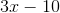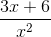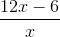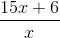Correct answer:Explanation:

Since both expressions have a common denominator, x2, we can just recopy the denominator and focus on the numerators. We get (9x - 2) - (6x - 8). We must distribute the negative sign over the 6x - 8 expression which gives us 9x - 2 - 6x + 8 ( -2 minus a -8 gives a +6 since a negative and negative make a positive). The numerator is therefore 3x + 6.

### Example Question #281 : Algebra

Simplify the following rational expression: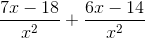Possible Answers: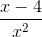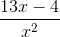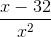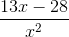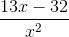Correct answer:Explanation:

Since both fractions in the expression have a common denominator of, we can combine like terms into a single numerator over the denominator: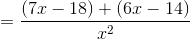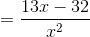### Example Question #14 : Expressions

Simplify the following expression: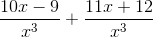Possible Answers: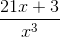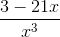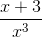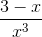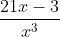Correct answer:Explanation:

Since both terms in the expression have the common denominator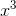, combine the fractions and simplify the numerators: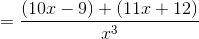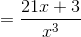### Example Question #13 : Expressions

Simplify the following rational expression: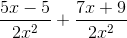Possible Answers: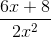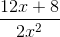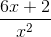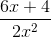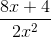Correct answer:Explanation:

Since both rational terms in the expression have the common denominator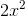, combine the numerators and simplify like terms: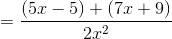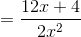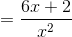### Example Question #281 : Algebra

Add and simplify: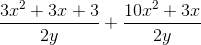Possible Answers: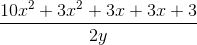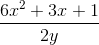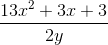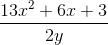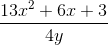Correct answer:Explanation:

When adding rational expressions with common denominators, you simply need to add the like terms in the numerator.

Therefore,is the best answer.

### All GRE Math Resources